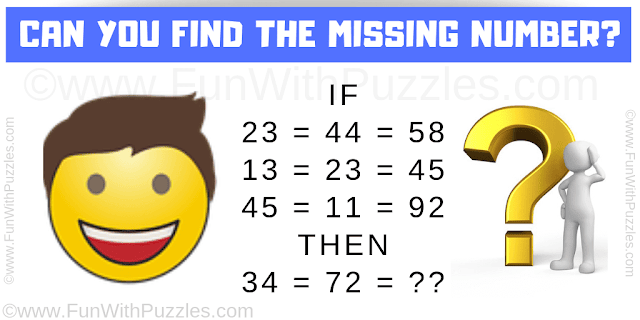This is quite an interesting maths logical question which will twist your mind. Solving this maths logic puzzle will help you to improve your logical reasoning. In this maths logical question, you are shown some number equation. Decode the logic in these equations to find the value of the missing number which will replace the question mark.Can you solve this Maths Logical Question?

Answer of this "Maths Logical Question", can be viewed by clicking on the answer button.

Unknown said...

79